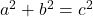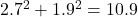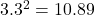Question

A triangle has side lengths of 1.9cm, 2.7cm and 3.3cm, all measured to 1 decimal place could this be a right angled triangle

1.Latifah

Yes

Step-by-step explanation:

A triangle is a “right” triangle if its lengths fullfil the Pythagorean theorem:Here, a and b are the shorter “legs” of the triangle, adjacent to its right angle, while c is the “hypotenuse,” the longest side and the one opposite the right angle.

If we call the 1.9 cm and 2.7 cm our legs, the sum of their squares is:cm

While squaring our hypotenuse gives uscm

The fact that we measured the triangle’s side lengths to 1 decimal point of accuracy means that there’s a little margin of error baked into the measurement. The two previous calculations are within 0.01 cm of each other, which is more than close enough, so we can say that yes, this could be a right triangle.